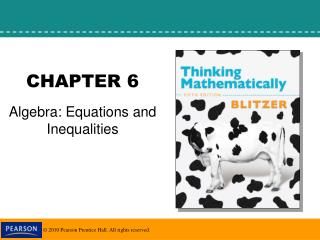DownloadDownload PresentationCHAPTER 6

# CHAPTER 6

Télécharger la présentation## CHAPTER 6

- - - - - - - - - - - - - - - - - - - - - - - - - - - E N D - - - - - - - - - - - - - - - - - - - - - - - - - - -
##### Presentation Transcript

1. CHAPTER 6 Algebra: Equations and Inequalities

2. 6.3 Applications of Linear Equations

3. Objectives Use linear equations to solve problems. Solve a formula for a variable.

4. Strategy for Solving Word Problems Step 1 Read the problem carefully several times until you can state in your own words what is given and what the problem is looking for. Let x (or any variable) represent one of the quantities in the problem. Step 2 If necessary, write expressions for any other unknown quantities in the problem in terms of x. Step 3 Write an equation in x that models the verbal conditions of the problem. Step 4 Solve the equation and answer the problem’s question. Step 5 Check the solution in the original wording of the problem, not in the equation obtained from the words.

5. Algebraic Translations of English Phrases

6. Example 1: Education Pays Off This graph shows the average yearly earnings in the United States by highest educational attainment.The average yearly salary of a man with an associate degree exceeds that of a man with some college by \$3 thousand. The average yearly salary of a man with a bachelor’s degree or more exceeds that of a man with some college by \$41 thousand. Combined, three men with these educational attainments earn \$188 thousand. Find the average yearly salary of men with each of these levels of education.

7. Example 2: continued Step 1: Let x represent one of the unknown quantities. Let x = the average yearly salary of a man with some college. Step 2: Represent the other unknown quantities in terms of x. x + 3 = the average yearly salary of a man with an associate degree x + 41 = the average yearly salary of a man with a bachelor’s degree or more.

8. Example 2: continued Step 3: Write an equation in x that models the conditions. x + (x + 3) + (x + 41) = 188 Step 4: Solve the equation and answer the question.

9. Example 2: continued The average salary with some college = 48 The average salary with an associate degree = x + 3 = 48 + 3 = 51 The average salary with a bachelor’s degree or more = x + 41 = 48 + 41 = 89. Some college = \$48 thousand per year Associate degree = \$51 thousand Bachelor’s degree = \$89 thousand Step 5: Check the proposed solution in the wording of the problem. The solution checks.

10. The total price of an article purchased on a monthly deferred payment plan is described by the following formula: T is the total price, D is the down payment, p is the monthly payment, and m is the number of months one pays. Solve the formula for p. T – D = D – D + pm T – D = pm T – D = pm m m T – D = p m Example 6: Solving a Formula for One of its Variables